# Problem-2 (Variance analysis; journal entries)

The Exide company is a single product company that uses standard costing system to control its costs. The standard and actual costs data for the most recent month to produce one unit of product is given below: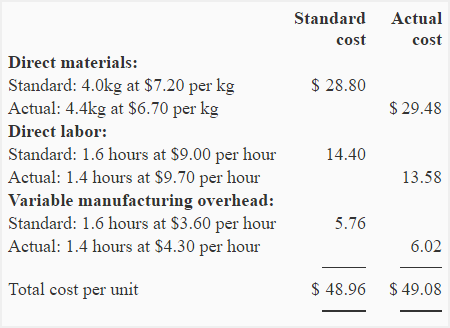During the most recent month, 4,800 units of product were produced. The comparison of standard and actual cost on the basis of total cost is given below: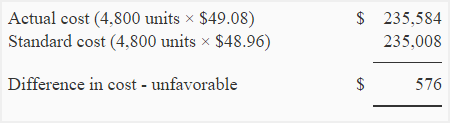During the month, the company purchased 21,120 kilograms of materials from its vendors. There was no inventory of materials in stock at the start and at the end of month.

Required:

1. (a) Compute direct materials price and quantity variances.
(b) Make journal entries to record direct materials related activities during the month.
2. (a) Compute direct labor rate and efficiency variances.
(b) Make journal entry to record direct labor direct labor cost during the month.
3. Compute variable manufacturing overhead spending and efficiency variances.
4. The total cost variance of \$576 is only 0.25% of \$235,008 standard cost which means the company’s costs are well under control. Do you agree? Explain.
5. What are possible causes of variances that you have computed in part 1, 2 and 3.

## Solution:

### (1) Variances and journal entries relating to direct materials:

a. Materials price variance:

(Actual quantity purchased × Actual price) – (Actual quantity purchased × Standard price)

= (21,120 kg × \$ 6.7) – (21,120 kg × \$ 7.2)

= \$141,504 – \$152,064

= \$10,560 Favorable

b. Materials quantity variance:

(Actual quantity used × Standard price) – (Standard quantity allowed × Standard price)

= (21,120 kg × \$7.2) – (*19,200 kg × \$7.2)

= \$152,064 – \$138,240

= \$13,824 Unfavorable

*4,800 units × \$4.0

c. Journal entries: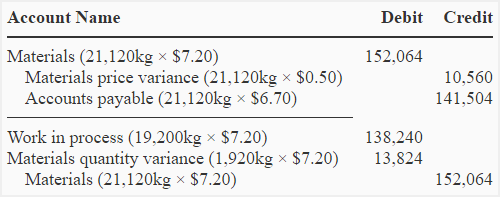### (2) Variances and journal entry relating to direct labor:

a. Labor rate variance:

(Actual hours worked × Actual rate) – (Actual hours worked × Standard rate)

= (6,720* × \$9.70) – (6,720 × \$9.00)

= \$65,184 – \$60,480

= \$4,704 Unfavorable

*4,800 units × 1.4 hours per unit

b. Labor efficiency variance:

(Actual hours worked × Standard rate) – (Standard hours allowed × Standard rate)

= (6,720 hours × \$9) – (7,680* hours × \$9)

= \$60,480 – \$69,120

= \$8,640 Favorable

*4,800 units × 1.6 hours per unit

c. Journal entry: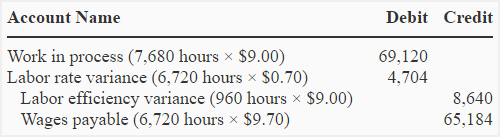### (3) Variable manufacturing overhead variances:

a. Variable overhead spending variance:

(Actual hours worked × Actual rate) – (Actual hours worked × Standard rate)

= (6,720 hours × \$4.3) – (6,720 hours × \$3.6)

= \$28,896 – \$24,192

= \$4,704 Unfavorable

b. Variable overhead efficiency variance:

(Actual hours worked × Standard rate) – (Standard hours allowed × Standard rate)

= (6,720 hours × \$3.6) – (7,680 hours × \$3.6)

= \$24,192 – \$27,648

= \$3,456 Favorable

### (4). Do you agree that costs are well under control?

The answer to requirement 4 is ‘no’. Although the total unfavorable cost variance of \$576 is only 0.25% of the total standard cost, it is made up of many individual variances and some of them may be large enough to require immediate investigation. A summary of variances computed in part 1, 2 and 3 is given below: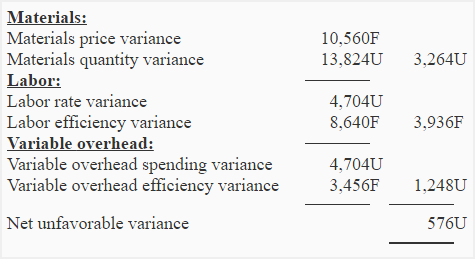### (5) Reasons of variances:

There are many causes of materials, labor and overhead variances. Some usual causes of the variances computed in part 1, 2 and 3 of this problem are given below:

1. Favorable direct materials price varianceDecrease in price of raw materials, discount for bulk purchase, purchase of low grade materials, inaccuracy in setting direct materials standards etc.
2. Unfavorable direct materials quantity variance: Unskilled or untrained workers, use of inferior quality materials, faulty or poorly adjusted machines and equipments, lack of care in using materials, inaccurate standards etc.
3. Unfavorable labor rate variance: Overtime, appointment of highly skilled workers, change in wage rates, inaccurate standards etc.
4. Favorable labor efficiency variance: Use of new and properly adjusted machines and equipments, appointment of highly skilled workers, use of high quality materials, inaccuracy in setting standards.
5. Unfavorable variable overhead spending variance: Increase in costs, purchase in uneconomical lots, spoilage, wastage, theft, inaccurate standards etc.
6. Favorable variable overhead efficiency variance: Use of new and properly adjusted machines and equipments, appointment of highly skilled workers, use of high quality materials, inaccuracy in setting standards. Notice that the causes of favorable variable overhead efficiency variance are same as that of favorable direct labor efficiency variance.
Show your love for us by sharing our contents.

### 3 Comments on Problem-2 (Variance analysis; journal entries)

1.Eric Rasmussen

Hi,

I know that the “variance” accounts (Direct Materials Price Variance, etc.) are equity accounts and when they have a credit (favorable) balance they are treated as revenues (or contra expenses), and when they have a debit (unfavorable) balance they are treated as expenses (or contra revenues), but what type of account are they? Contra COGS? Are they a category of their own? I can’t seem to find a resource that directly says what they are.

Thanks,

Eric Rasmussen
Instructor
Pioneer Pacific College
Springfield, OR

2.Sminder Bhinder

2b) 2b is labeled wrong .It should be labor Efficiency variance and not labor rate variance

3.FEDRIGO

STOCK IN BOOKS 120 SQM / STOCK IS STORE 100 SQM

WHATS IS ACCOUNTING ENTRY TO ADJUST THE STOCK IN BOOKS